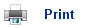# Electrochemical Cells

• An electrochemical cell can allow two half-reactions to occur separately, with electrons flowing through an external wire, from one reaction to the other.
• This is the basis in all batteries and “dry” cells.
• In one part of the cell, the oxidation reaction is taking place; in the other part, the reduction reaction is occurring.
• The electrons that have been lost in the oxidation reaction travel through the external circuit to the reduction reaction, where they reduce the oxidising agent.
• The two parts of the electrochemical cell are called half-cells.
• The energy is given out as electrical energy instead of heat.
• Batteries have both a positive and negative terminal; the potential difference between the positive and negative terminals is measured in volts.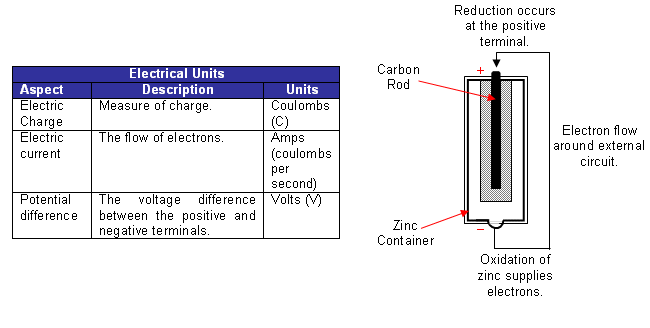• The voltage can also be expressed as the number of joules of energy transferred when one coulomb of charge flows around the circuit:

1 volt = 1 J C-1

• For example, when 1 coulomb of charge flows through a potential difference of 3 volts, 3J of energy are transferred. After an amount of time, the voltage in a battery can drop; this is due to the current; the higher the current drawn, the lower the voltage the battery may give. Thus, when comparing cells and half-cells, it is important that the potential difference is measured when no current flows. This potential difference is given the symbol Ecell (sometimes referred to as electromotive force, or emf, even though it’s not actually a force).
• A high resistance voltmeter is used to measure the potential difference between the electrodes of the two half-cells. It is high resistance, so that negligible current flows, and therefore the maximum potential difference is being measured.
• From the measured potential differences of half-cells, it is possible to work out a scale of individual electrode potentials.
• This sort of scale can be used to tell us, for any pair of half-cells, which one would be the positive electrode.
• Electrons flow to the half-cell with the more positive potential and cause a reduction reaction; an oxidation reaction must occur in the other half-cell.
• Therefore, using a scale of electrode potentials, it is possible to determine what is oxidised and what is reduced in a Redox reaction.

## Metal- metal ion cells

• A simple cell can be established by using strips of metal submersed into solutions of metal ions; for example, the copper and zinc cell consists of two half-cells: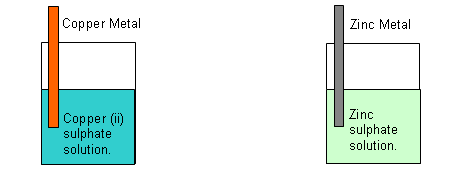• A connection is needed between the two solutions (the solutions should not mix together).
• A strip of filter paper soaked in saturated potassium nitrate can be used as a junction between the two half-cells (salt bridge). The salt bridge contains both positive and negative ions. Whichever half-cell becomes more negative (i.e. contains the oxidising agent) will receive positive ions from the salt bridge, cancelling the charge. The half-cell that becomes more positive (i.e. the one containing the reducing agent) will receive negative ions from the saltbridge, cancelling the charge.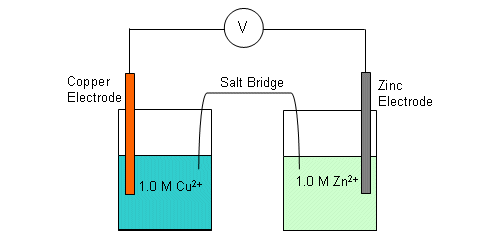• In a half-cell, there is an equilibrium reaction occurring at the surface of the electrode; for example in the zinc half-cell:

Zn(s)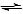Zn2+(aq) + 2e

• When the two half-cells are connected together, the species that is most reactive will have the greatest tendency for it to form ions i.e. become the reducing agent.
• When the zinc half-cell is connected to the copper half-cell; the copper ions will accept electrons, as they are the stronger oxidising agent. This will shift the position of equilibrium in the copper half-reaction to the right, thus resulting in the reduction of copper ions.

Cu (s)Cu2+(aq) + 2e

• A high resistance voltmeter is connected between the copper and zinc electrodes. The table below shows the data from a number of different cells: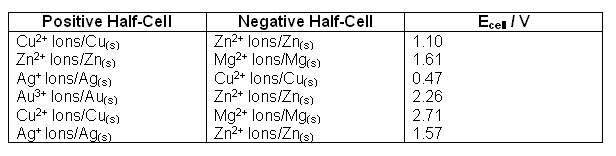• It is hard to detect any patterns in the voltages; different pairs of half-cells are giving almost the same voltage in some cases.
• One way of sorting out some of the data is show below.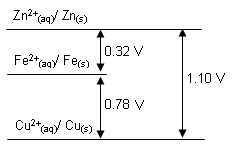• The potential difference between the copper and iron half-cells is 0.78V. The potential difference between the iron and zinc half-cells is 0.32V (iron the positive half-cell). Therefore the difference between the zinc and copper half-cells must be 1.10V.
• A hydrogen half-cell is used as a standard reference; i.e. the hydrogen half-cell is used to construct a list of electrode potentials.• Standard conditions are used, so as not to alter the voltage (voltage changes with temperature).
• The potential of the hydrogen half-cell is defined as 0V.
• The standard electrode potential of a half cell,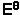, is defined as the potential difference between it and a standard hydrogen half-cell.
• Some values are shown below: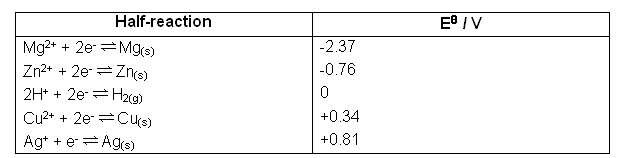• The table above is called the electrochemical series.
• The metal with the most negative electrode potential has the highest tendency to act as a reducing agent, supplying electrons when it is oxidised.
• The metal with the least negative electrode potential has a much lower tendency to act as a reducing agent.
• The table can be used to allow us to calculate the maximum voltage obtainable from any standard cell.
• It can also be used to make predictions about any Redox reaction, and whether or not it will occur in a cell.
• The electrochemical series can be found in your data book.

## Other half-cell reactions

• In addition to just metal/metal ion reactions, there are also many other kinds of half-cell reactions, for example:

Between ions:

Fe3+ (aq) + e-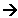Fe2+ (aq)
Cr2O72- (aq) + 14H+(aq) + 6e-2Cr3+ (aq) + 7H2O (l)
MnO4- (aq) + 8H+(aq) + 5e-Mn2+ (aq) + 4H2O (l)

Between molecules and ions:

Cl2(aq) + 2e-2Cl- (aq)

• With half-cells like these, electrical contact is made with an inert electrode dipping into the solution containing all the ions and molecules involved in the redox half-reaction.

## The Electrochemical Series

• The more positive the value of a species in the series, the stronger the oxidising agent.
• From the electrochemical series, you are able to work out in which direction the half-cell reaction will occur.
• The electrode potential is a measure of the tendency of a half-reaction to supply electrons:
• Zinc Metal in a zinc solution has an electrode potential of -0.76V, and so has a high tendency to supply electrons.
• Copper metal in a copper solution has an electrode potential of +0.34V, and so has a lower tendency to supply electrons.
• Silver metal in a silver solution has an electrode potential of +0.80V and so has a very low tendency to supply electrons.
• So, zinc can supply electrons to copper, which can supply electrons to silver, but the reverse cannot occur.
• For zinc to supply electrons, the reaction must be:

Zn(s)Zn2+(aq) + 2e-

• For copper to accept electrons, the reaction must be:

Cu2+(aq) + 2e-Cu(s)

• The prediction for the overall reaction agrees with the observed changes:

Zn(s) + Cu2+Zn2+ + Cu(s)

• The highest electrode potential in two connected half-cells is always the positive electrode of a cell. Electrode potential charts provide a useful way of displaying and using the electrochemical series data: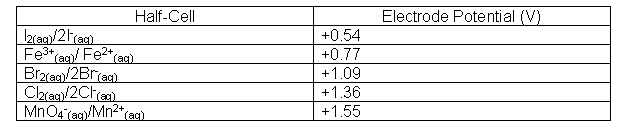## Using Electrode Potential Charts

Example Question:

The table below gives electrode potentials of some half-reactions. What reactions can occur if we connect the MnO4-(aq)/Mn2+(aq) and Fe3+(aq) half-cells, so that electrons can flow? Write an equation for the overall reaction.Method:

1. Construct an electrode potential chart.
2. Use it to make predictions about half-reactions.
3. Balance the half-equations.
1.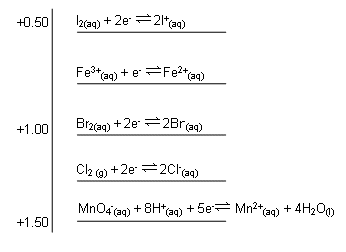2. Electrons flow to the positive electrode of a cell; this will be to the MnO4- (aq) /Mn2+(aq) half-cell (this is more positive than the Fe3+(aq) / Fe2+(aq) half-cell.

A reduction reaction will occur at the positive electrode:

MnO4-(aq) + 8H+(aq) + 5e-Mn2+(aq) + 4H2O(l)

The other half-cell will supply the electrons:

Fe2+(aq)Fe3+(aq) + e-

3. Now to balance the equations to make sure they match in number of electrons:

5Fe2+(aq)5Fe3+(aq) + 5e-

The overall reaction will be:

MnO4-(aq) + 8H+(aq) + 5Fe2+(aq)Mn2+(aq) + 4H2O(l) + 5Fe3+(aq)

## Using electrode potentials to predict reactions

• Electrode potentials can be used to make predictions about any redox reaction.
• However, these are only predictions, and therefore are not certainty.
• Electrode potentials can only be used to decide whether a reaction is possible; even if it is possible it may be too slow to actually occur.
• If a reaction is slow, it is often because the activation energy is too low.
• So, even if the electrode potentials state that a reaction will occur; in reality the activation energy is too high for it to work.
• We could make the reaction work by adding a catalyst, which lowers the activation energy, or by changing the conditions (i.e. temperature, pressure etc.).
• If the electrode potentials state that a reaction cannot occur, then the reaction will never occur, no matter what is done to try and make it happen.

## Useful books for revision

Revise A2 Chemistry for Salters (OCR A Level Chemistry B)Salters (OCR) Revise A2 ChemistryHome